# Lazy Dynamic Programming

Dynamic programming is a method for efficiently solving complex problems with overlapping subproblems, covered in any introductory algorithms course. It is usually presented in a staunchly imperative manner, explicitly reading from and modifying a mutable array—a method that doesn’t neatly translate to a functional language like Haskell.

Happily, laziness provides a very natural way to express dynamic programming algorithms. The end result still relies on mutation, but purely by the runtime system—it is entirely below our level of abstraction. It’s a great example of embracing and thinking with laziness.

So let’s look at how to do dynamic programming in Haskell and implement string edit distance, which is one of the most commonly taught dynamic programming algorithms. In a future post, I will also extend this algorithm to trees.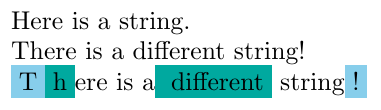The differences between two strings, as computed by the edit distance algorithm.

This post was largely spurred on by working with Joe Nelson as part of his “open source pilgrimage”. We worked on my semantic version control project which, as one of its passes, needs to compute a diff between parse trees with an algorithm deeply related to string edit distance as presented here. Pairing with Joe really helped me work out several of the key ideas in this post, which had me stuck a few years ago.

## Dynamic Programming and Memoization

Dynamic programming is one of the core techniques for writing efficient algorithms. The idea is to break a problem into smaller subproblems and then save the result of each subproblem so that it is only calculated once. Dynamic programming involves two parts: restating the problem in terms of overlapping subproblems and memoizing.

Overlapping subproblems are subproblems that depend on each other. This is where dynamic programming is needed: if we use the result of each subproblem many times, we can save time by caching each intermediate result, only calculating it once. Caching the result of a function like this is called memoization.

Memoization in general is a rich topic in Haskell. There are some very interesting approaches for memoizing functions over different sorts of inputs like Conal Elliott’s elegant memoization or Luke Palmer’s memo combinators.

The general idea is to take advantage of laziness and create a large data structure like a list or a tree that stores all of the function’s results. This data structure is defined circularly: recursive calls are replaced with references to parts of the data structure. Thanks to laziness, pieces of the data structure only get evaluated as needed and at most once—memoization emerges naturally from the evaluation rules. A very illustrative (but slightly cliche) example is the memoized version of the Fibonacci function:

fib n = fibs !! n
where fibs = 0 : 1 : zipWith (+) fibs (drop 1 fibs)

The fib function indexes into fibs, an infinite list of Fibonacci numbers. fibs is defined in terms of itself : instead of recursively calling fib, we make later elements of fibs depend on earlier ones by passing fibs and (drop 1 fibs) into zipWith (+). It helps to visualize this list as more and more elements get evaluated:

•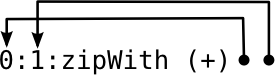•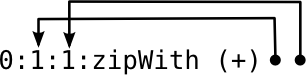•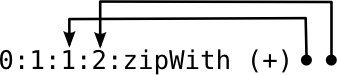•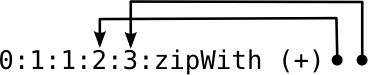•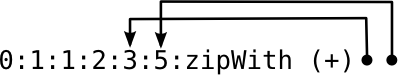•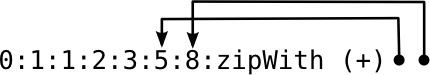zipWith f applies f to the first elements of both lists then recurses on their tails. In this case, the two lists are actually just pointers into the same list!

Note how we only ever need the last two elements of the list. Since we don’t have any other references to the fibs list, GHC’s garbage collector can reclaim unused list elements as soon as we’re done with them. So with GC, the actual execution looks more like this:

••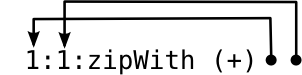•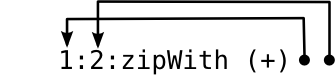•••More memory efficient: we only ever store a constant number of past results.

### Lazy Arrays

Dynamic programming algorithms tend to have a very specific memoization style—sub-problems are put into an array and the inputs to the algorithm are transformed into array indices. These algorithms are often presented in a distinctly imperative fashion: you initialize a large array with some empty value and then manually update it as you go along. You have to do some explicit bookkeeping at each step to save your result and there is nothing preventing you from accidentally reading in part of the array you haven’t set yet.

This imperative-style updating is awkward to represent in Haskell. We could do it by either passing around an immutable array as an argument or using a mutable array internally, but both of these options are unpleasant to use and the former is not very efficient.

Instead of replicating the imperative approach directly, we’re going to take advantage of Haskell’s laziness to define an array that depends on itself. The trick is to have every recursive call in the function index into the array and each array cell call back into the function. This way, the logic of calculating each value once and then caching it is handled behind the scenes by Haskell’s runtime system. We compute the subproblems at most once in the order that we need and the array is always used as if it was fully filled out: we can never accidentally forget to save a result or access the array before that result has been calculated.

At its heart, this is the same idea as having a fibs list that depends on itself, just with an array instead of a list. Arrays fit many dynamic programming problems better than lists or other data structures.

We can rewrite our fib function to use this style of memoization. Note that this approach is actually strictly worse for Fibonacci numbers; this is just an illustration of how it works.

fib' max = go max
where go 0 = 0
go 1 = 1
go n = fibs ! (n - 1) + fibs ! (n - 2)
fibs = Array.listArray (0, max) [go x | x <- [0..max]]

The actual recursion is done by a helper function: we need this so that our memoization array (fibs) is only defined once in a call to fib' rather than redefined at each recursive call!

For calculating fib' 5, fibs would be an array of 6 thunks each containing a call to go. The final result is the thunk with go 5, which depends on go 4 and go 3; go 4 depends on go 3 and go 2 and so on until we get to the entries for go 1 and go 0 which are the base cases 1 and 0.

The nice thing is that this tangle of pointers and dependencies is all taken care of by laziness. We can’t really mess it up or access the array incorrectly because those details are below our level of abstraction. Initializing, updating and reading the array is all a result of forcing the thunks in the cells, not something we implemented directly in Haskell.

## String Edit Distance

Now that we have a neat technique for dynamic programming with lazy arrays, let’s apply it to a real problem: string edit distance. This is one of the most common examples used to introduce dynamic programming in algorithms classes and a good first step towards implementing tree edit distance.

The edit distance between two strings is a measure of how different the strings are: it’s the number of steps needed to go from one to the other where each step can either add, remove or modify a single character. The actual sequence of steps needed is called an edit script. For example:

• "brother""bother"    remove 'r'
• "bother"   → "brother"  add 'r'
• "sitting""fitting"  modify 's' to 'f'

The distance between strings $$a$$ and $$b$$ is always the same as the distance between $$b$$ and $$a$$. We go between the two edit scripts by inverting the actions: flipping modified characters and interchanging adds and removes.

The Wagner-Fischer algorithm is the basic approach for computing the edit distance between two strings. It goes through the two strings character by character, trying all three possible actions (adding, removing or modifying) and picking the action that minimizes the distance.

For example, to get the distance between "kitten" and "sitting", we would start with the first two characters k and s. As these are different, we need to try the three possible edit actions and find the smallest distance. So we would compute the distances between "itten" and "sitting" for a delete, "kitten" and "itting" for an insert and "itten" and "itting" for a modify, and choose the smallest result.

This is where the branching factor and overlapping subproblems come from—each time the strings differ, we have to solve three recursive subproblems to see which action is optimal at the given step, and most of these results need to be used more than once.

We can express this as a recurrence relation. Given two strings $$a$$ and $$b$$, $$d_{ij}$$ is the distance between their suffixes of length $$i$$ and $$j$$ respectively. So, for "kitten" and "sitting", $$d_{6,7}$$ would be the whole distance while $$d_{5,6}$$ would be between "itten" and "itting".

\begin{align} d_{i0} & = i & \text{ for } 0 \le i \le m & \\ d_{0j} & = j & \text{ for } 0 \le j \le n & \\ d_{ij} & = d_{i-1,j-1}\ & \text{if } a_i = b_j & \\ d_{ij} & = \min \begin{cases} d_{i-1,j} + 1\ \ \ \ (\text{delete}) \\ d_{i,j-1} + 1\ \ \ \ (\text{insert}) \\ d_{i-1,j-1} + 1\ (\text{modify}) \\ \end{cases} & \text{if } a_i \ne b_j \end{align}

The base cases $$d_{i0}$$ and $$d_{0j}$$ arise when we’ve gone through all of the characters in one of the strings, since the distance is just based on the characters remaining in the other string. The recursive case has us try the three possible actions, compute the distance for the three results and return the best one.

We can transcribe this almost directly to Haskell:

naive a b = d (length a) (length b)
where d i 0 = i
d 0 j = j
d i j
| a !! (i - 1) ==  b !! (j - 1) = d (i - 1) (j - 1)
| otherwise = minimum [ d (i - 1) j       + 1
, d i (j - 1)       + 1
, d (i - 1) (j - 1) + 1
]

And, for small examples, this code actually works! You can try it on "kitten" and "sitting" to get 3. Of course, it runs in exponential time, which makes it freeze on larger inputs—even just "aaaaaaaaaa" and "bbbbbbbbbb" take a while! The practical version of this algorithm needs dynamic programming, storing each value $$d_{ij}$$ in a two-dimensional array so that we only calculate it once.

We can do this transformation in much the same way we used the fibs array: we define ds as an array with a bunch of calls to d i j and we replace our recursive calls d i j with indexing into the array ds ! (i, j).

basic a b = d m n
where (m, n) = (length a, length b)
d i 0 = i
d 0 j = j
d i j
| a !! (i - 1) ==  b !! (j - 1) = ds ! (i - 1, j - 1)
| otherwise = minimum [ ds ! (i - 1, j)     + 1
, ds ! (i, j - 1)     + 1
, ds ! (i - 1, j - 1) + 1
]

ds = Array.listArray bounds
[d i j | (i, j) <- Array.range bounds]
bounds = ((0, 0), (m, n))

This code is really not that different from the naive version, but far faster.

### Faster Indexing

One thing that immediately jumps out from the above code is !!, indexing into lists. Lists are not a good data structure for random access! !! is often a bit of a code smell. And, indeed, using lists causes problems when working with longer strings.

We can solve this by converting a and b into arrays and then indexing only into those. (We can also make the arrays 1-indexed, simplifying the arithmetic a bit.)

better a b = d m n
where (m, n) = (length a, length b)
a'     = Array.listArray (1, m) a
b'     = Array.listArray (1, n) b

d i 0 = i
d 0 j = j
d i j
| a' ! i ==  b' ! j = ds ! (i - 1, j - 1)
| otherwise = minimum [ ds ! (i - 1, j)     + 1
, ds ! (i, j - 1)     + 1
, ds ! (i - 1, j - 1) + 1
]

ds = Array.listArray bounds
[d i j | (i, j) <- Array.range bounds]
bounds = ((0, 0), (m, n))

The only difference here is defining a' and b' and then using ! instead of !!. In practice, this is much faster than the basic version.

Note: I had a section here about using lists as loops which wasn’t entirely accurate or applicable to this example, so I’ve removed it.

### Final Touches

Now we’re going to do a few more changes to make our algorithm complete. In particular, we’re going to calculate the edit script—the list of actions to go from one string to the other—along with the distance. We’re also going to generalize our algorithm to support different cost functions which specify how much each possible action is worth.

The first step, as ever, is to come up with our data types. How do we want to represent edit scripts? Well, we have four possible actions:

data Action = None | Add | Remove | Modify

We’ll also take an extra argument, the cost function, which makes our final function type:

script :: Eq a => (Action -> Distance) -> [a] -> [a] -> [Action]

We could calculate the action by traversing our memoized array, seeing which action we took at each optimal step. However, for simplicity—at the expense of some performance—I’m just going to put the script so far at each cell of the array. Thanks to laziness, only the scripts needed for the end will be evaluated… but that performance gain is more than offset by having to store the extra thunk in our array.

The basic skeleton is still the same. However, we need an extra base case: d 0 0 is now special because it’s the only time we have an empty edit script. We extract the logic of managing the edit scripts into a helper function called go. At each array cell, I’m storing the score and the list of actions so far: (Distance, [Action]). Since the script is build up backwards, I have to reverse it at the very end.

script cost a b = reverse . snd \$ d m n
where (m, n) = (length a, length b)
a'     = Array.listArray (1, m) a
b'     = Array.listArray (1, n) b

d 0 0 = (0, [])
d i 0 = go (i - 1) 0 Remove
d 0 j = go 0 (j - 1) Add
d i j
| a' ! i ==  b' ! j = go (i - 1) (j - 1) None
| otherwise = minimum' [ go (i - 1) j       Remove
, go i (j - 1)       Add
, go (i - 1) (j - 1) Modify
]

minimum' = minimumBy (comparing fst)
go i j action = let (score, actions) = ds ! (i, j) in
(score + cost action, action : actions)

ds = Array.listArray bounds [d i j | (i, j) <- Array.range bounds]
bounds = ((0, 0), (m, n))

The final piece is explicitly defining the old cost function we were using:

cost :: Action -> Distance
cost None = 0
cost _    = 1

You could also experiment with other cost functions to see how the results change.

We now have a very general technique for writing dynamic programming problems. We take our recursive algorithm and:

1. add an array at the same scope level as the recursive function
2. define each array element as a call back into the function with the appropriate index
3. replace each recursive call with an index into the array

This then maintains all the needed data in memory, forcing thunks as appropriate. All of the dependencies between array elements—as well as the actual mutation—is handled by laziness. And, in the end, we get code that really isn’t that far off from a non-dynamic recursive version of the function!

For a bit of practice, try to implement a few other simple dynamic programming algorithms in Haskell like the longest common substring algorithm or CYK parsing.